﻿ L_WindowLevelFillLUTExt2 | Function References | Raster Imaging C API Help
In This Topic ▼

# L_WindowLevelFillLUTExt2

#include "l_bitmap.h"

L_LTDIS_API L_INT L_WindowLevelFillLUTExt2(pLUT, ulLUTLen, pRgbStart, pRgbEnd, nLow, nHigh, uLowBit, uHighBit, nMinValue, nMaxValue, nFactor, uFlags)

Fills the user-allocated 16-bit LUT with values ranging between the `pRgbStart` and `pRgbEnd` colors, according to the selected LUT type.

## Parameters

Pointer to an array to be updated with the RGB quad (i.e., the lookup table).

### L_UINT ulLUTLen

The size of `pLUT`. The minimum size is 2 raised to the power of (`uHighBit` - `uLowBit` + 1).

Starting color value for the gradient.

Ending color value for the gradient.

### L_INT nLow

The low value of the window width, in pixels.

### L_INT nHigh

The high value for the window width, in pixels.

### L_UINT uLowBit

Value indicating the low bit used for leveling. This is normally 0 and should be less than the uHighBit.

### L_UINT uHighBit

Value indicating the high bit used for leveling. This should be greater than or equal to uLowBit and less than 11 for 12-bit grayscale or 15 for 16-bit grayscale.

### L_INT nMinValue

The bitmap's minimum value. This value can be obtained by calling L_GetMinMaxVal.

### L_INT nMaxValue

The bitmap's maximum value. This value can be obtained by calling L_GetMinMaxVal.

### L_INT nFactor

Value that indicates the factor to be applied in the function operation specified in the uFlags parameter. This parameter is used only if `uFlags` is FILLLUT_EXPONENTIAL, FILLLUT_LOGARITHMIC or FILLLUT_SIGMOID. If FILLLUT_EXPONENTIAL or FILLLUT_SIGMOID flag is selected, its value can be any integer (+/-). If FILLLUT_LOGARITHMIC flag is selected, its value should be >= 0. If nFactor = 0, the lookup table will be filled linearly.

### L_UINT uFlags

Flags that indicate how the range is used to fill the LUT and the type of LUT and whether the LUT contains signed or unsigned data. The following flags indicate how the range is used to fill the LUT:

Value Meaning
FILLLUT_INSIDE [0x0001] Fill the LUT with values between nLow and nHigh (inside the range[nLow,nHigh])
FILLLUT_OUTSIDE [0x0002] Fill the LUT below `nLow` and above `nHigh` and also in-between.

#### The following flags indicate the LUT type:

Value Meaning
FILLLUT_LINEAR [0x0010] The LUT is linear.
FILLLUT_EXPONENTIAL [0x0020] The LUT is exponential.
FILLLUT_LOGARITHMIC [0x0030] The LUT is logarithmic.
FILLLUT_SIGMOID [0x0040] The LUT is sigmoid.

#### The following flags indicate whether the LUT contains signed or unsigned data:

Value Meaning
FILLLUT_UNSIGNED [0x0000] The LUT data is unsigned.
FILLLUT_SIGNED [0x0100] The LUT data is signed.

#### The following flag indicates whether to fill the LUT using only the `pRgbStart` and `pRgbEnd` colors, as is used in DICOM applications:

Value Meaning
FILLLUT_DICOM_STYLE [0x1000] Fill using only the pRgbStart and pRgbEnd colors.

#### Returns

Value Meaning
SUCCESS The function was successful.
< 1 An error occurred. Refer to Return Codes.

Use L_WindowLevelFillLUTExt2 to fill in a 16-bit LUT used by L_WindowLevelExt or L_WindowLevelBitmapExt, according to the LUT type flag. To fill an 8-bit LUT, call L_WindowLevelFillLUT.

L_WindowLevelFillLUTExt and L_WindowLevelFillLUTExt2 are very similar. The methods have the following differences:

1. L_WindowLevelFillLUTExt2 does not check the FILLLUT_DICOM_STYLE flag: it assumes it is always TRUE (fill the LUT using only the `pRgbStart` and `pRgbEnd` colors). Use L_WindowLevelFillLUTExt if the LUT needs to be filled a different way.
2. L_WindowLevelFillLUTExt2 does not assume that the “low” and “high” values have already been shifted by the “LowBit”. L_WindowLevelFillLUTExt does.

L_WindowLevelFillLUTExt2 fills the LUT as follows:

Inside/Outside nMinValue-nLow nLow-nHigh nHigh-nMaxValue
FILLLUT_INSIDE Solid black (0,0,0) Color gradient ranging from rgbStart to rgbEnd Solid white (0xFFFF, 0xFFFF, 0xFFFF)
FILLLUT_OUTSIDE Solid color (rgbStart) Grayscale values from solid black (0,0,0) to solid white (0xFFFF, 0xFFFF, 0xFFFF) Solid color (rgbEnd)
FILLLUT_INSIDE | FILLLUT_DICOM_STYLE None Color gradient ranging from rgbStart to rgbEnd None
FILLLUT_OUTSIDE | FILLLUT_DICOM_STYLE Solid color (rgbStart) Color gradient ranging from rgbStart to rgbEnd Solid color (rgbEnd)

The `nFactor` parameter is used for the logarithmic, exponential, and sigmoid functions only. If nFactor = 0, the function performs a linear interpolation between the two points `nLow` and `nHigh`, and stores the results in the lookup table, regardless of the value in `uFlags`.

If the uFlags parameter is FILLLUT_EXPONENTIAL, the value of nFactor modifies the lookup table values (see the figure below) according to the following equations: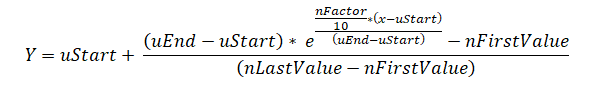where: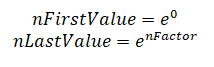x = the intensity value of the selected point

uStart = the nLow parameter of this function

uEnd = the nHigh parameter of this function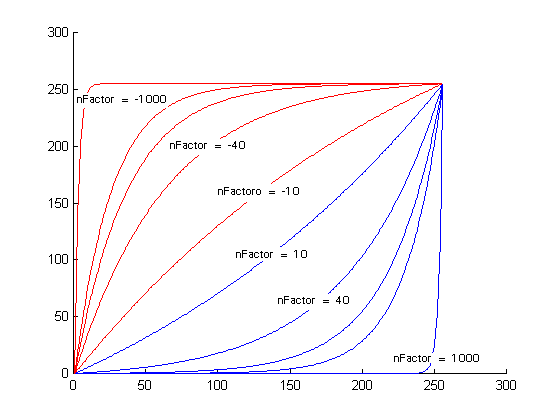If uFlags is FILLLUT_ LOGARITHMIC, the value of nFactor modifies the lookup table values (see the figure below) according to the following equations: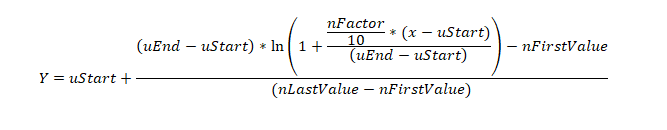where: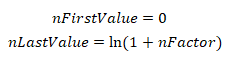x = the intensity value of the selected point

uStart = the nLow parameter of this function

uEnd = the nHigh parameter of this function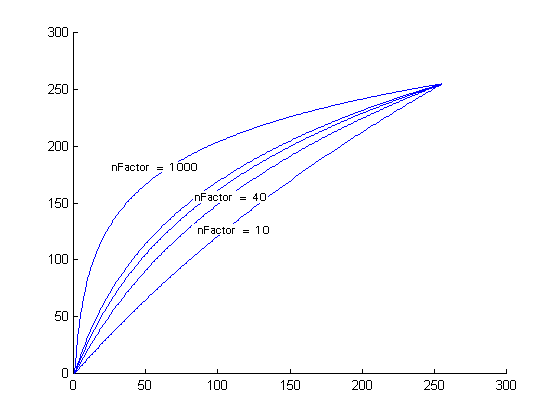If the uFlags parameter is FILLLUT_ SIGMOID, the value of nFactor modifies the lookup table values (see the figure below)according to the following equations: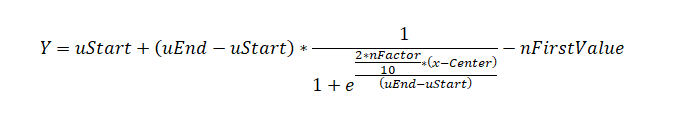where: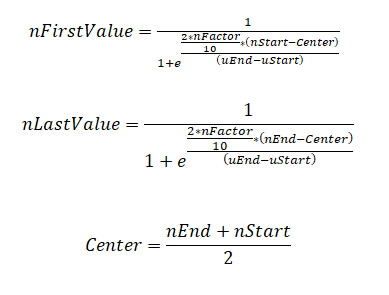x = the intensity value of the selected point

uStart = the nLow parameter of this function

uEnd = the nHigh parameter of this function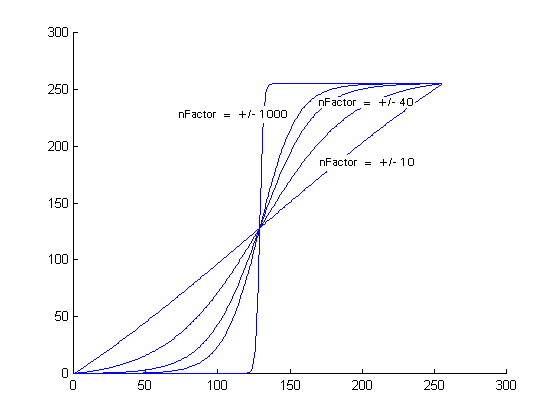If the uFlags parameter is FILLLUT_LINEAR, `nFactor` is ignored. The function fills the lookup table linearly.

Note: This function only works for 12- or 16-bit grayscale images. Trying to use this function with other images will result in an error.

Allocate the memory for the LUT before calling this function. Calculate the required size with (sizeof(RGBQUAD) * (1<<(pBitmap->HighBit - pBitmap->LowBit + 1))), as shown in the example below.

For example, suppose you are working with a 12-bit grayscale image. There are 4096 intensity levels in a 12-bit image (2 raised to the 12th power). Normally, the interval between 0 and 4095 for unsigned data would be mapped to colors between (0, 0, 0) and (0xFFFF, 0xFFFF, 0xFFFF). With this function, any value that falls between the low level and the high level will be mapped to colors between the starting color and the ending color. If you do not want a mapping function, set the starting and ending colors to the same value.

If you only want to map the values between 1972 and 3273 (in a 12-bit unsigned image), then set `nLow` to 1972, set `nHigh` to 3273, and set the FILLLUT_INSIDE option. To map the values less than 1972 and greater than 3273, select FILLLUT_OUTSIDE option.

LEADTOOLS supports two types of LUTs for 10-16-bit grayscale images: 8-bit LUT and 16-bit LUT.  Typical grayscale image display and processing is done using an 8-bit LUT. But, you can also use a 16-bit LUT, which offers more precision. Some special video cards and monitors also support the display of grayscale images using a 16-bit LUT.

For information about saving bitmaps that have been window-leveled, refer to Saving Window-Leveled Bitmaps.

Required DLLs and Libraries

Platforms

Win32, x64, Linux.

Help Version 20.0.2019.12.5